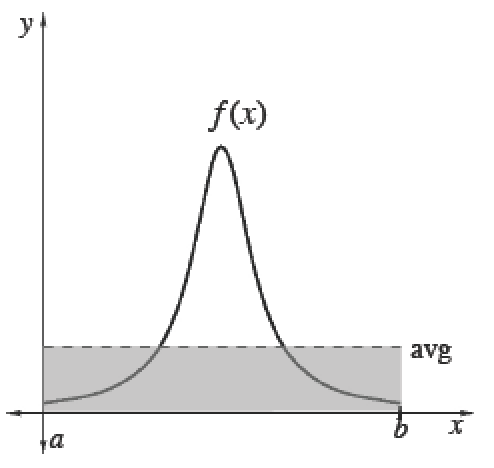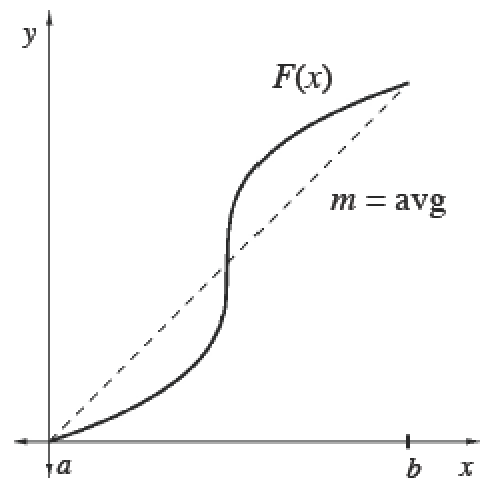### Home > APCALC > Chapter 7 > Lesson 7.3.1 > Problem7-106

7-106.

Multiple Choice: The average value of $f(x) = e^{\sin(x^2)}$ on the interval $−1 ≤ x ≤ 1$ is nearest to:

1. $−1.253$

1. $−1.414$

1. $0$

1. $1.253$

1. $1.414$

Do not confuse average value of $f$ with average rate of change of $f$.

Average (Mean) Values

 To calculate the mean (average) value of a finite set of items, add up the values of items and divide by the number of items.Integrals help us add over a continuous interval. Therefore, for any continuous function $f$ :$\frac{\int_a^bf(x)dx}{b-a}=$ mean value of $f$ over $[a, b]$Since $\int _ { a } ^ { b } f ( x ) d x$, we can also calculate the average value of any function $f$ using its antiderivative $F$. Its average slope gives the average rate of change of $F$, which is the same as the average value of $f.$$\frac{\int_a^bf(x)dx}{b-a}=\frac { F ( b ) - F ( a ) } { b - a }=$  mean rate of change of $F ′$ over $[a, b]$